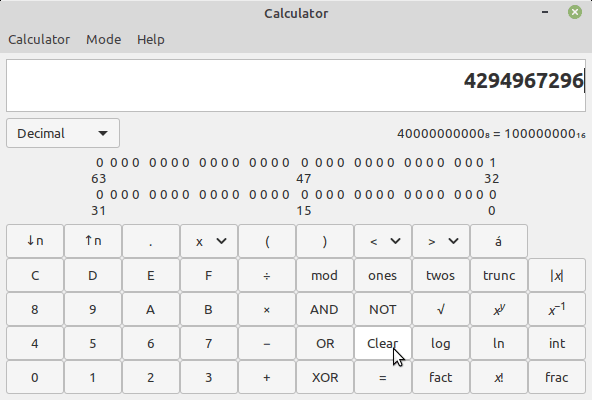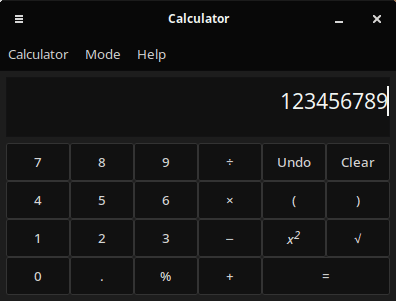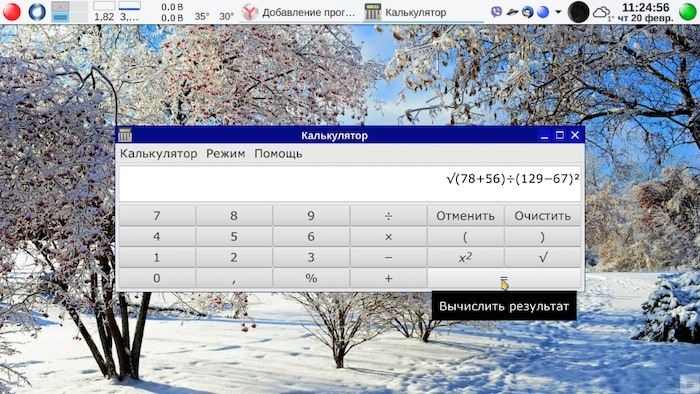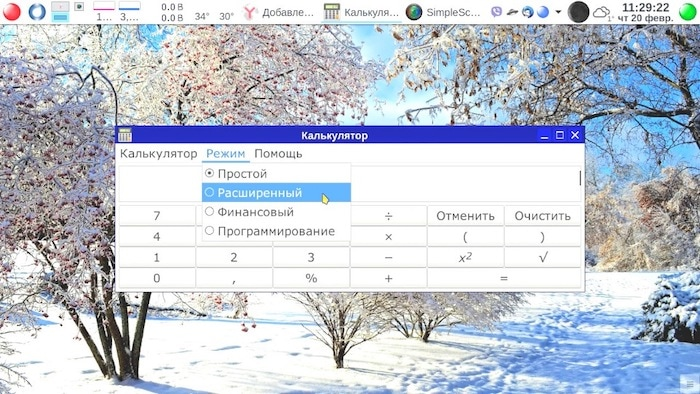# MATE Calculator

MATE calculator — the calculator used in the desktop environment MATE.

Calculator MATE (mate-calc) calculator is a fork of gnome-calc.

Opportunities

Four modes of operation:

• Basic
• Financial
• Programming

Displaying numbers in different formats:

• Automatic
• Fixed
• Scientific
• Engineering

The choice of word size (Word size):

• 8-bit
• 16-bit
• 32-bit
• 64-bit

The display angles:

• C

Development

A fork of gnome-calc

Programming language: C

Developed in the framework of the project of MATE (the desktop environment).

Calculator graphical environment MATE. Has some very useful features.

First, the supports brackets.
Second, it shows the input formula is based on your displaceme, allowing you to edit the judges. Staff gadowski calculator KCalc, alas, these functions are not supported. In fact, the problem faced, even the sounds of Windows. And at the same time working as a teacher in economic University. Proverai work of students, had in large numbers compute fractions with the operations of addition and/or subtraction in the numerator and the denominator .It was extremely uncomfortable – first calculate the numerator, once characterized, then the denominator, and then divide the first by the second. As you can see from the first screenshot, calculator MATE has no such drawback. Moreover, allows you to extend the functionality even in easy mode some common functions square and square root. In fairness, gnomes calculator also has (at least used to have) the same functionality, but in KDE it looks too shabby. Not because he’s so bad he’s just another graphical environment. Would, of course, sad, if not found calculator by MATE.
Of course, like any normal calculator, the subject has several modes with different functionality, although personally I’m in the 99% case, use the easiest. As you can see from the second screenshot, all four modes.

Install MATE Calculator in Ubuntu Linux:sudo apt install mate-calc

• MATE Calculator (Linux Mint 19.3) (version: 1.20.1)• MATE Calculator (distribution Solus 4.1) (version: 1.22.2)• MATE CalculatorСкриншот provided by the user.• MATE CalculatorСкриншот provided by the user.Source: pingvinus.ru

(Visited 6 times, 1 visits today)